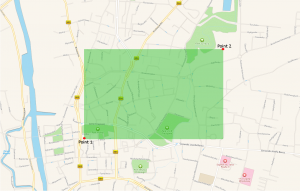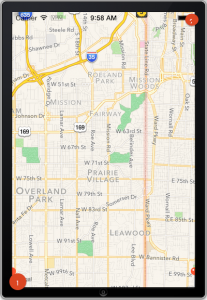# How to get geographic coordinates of the visible MKMapView area in iOS

## How to get geographic coordinates of the visible MKMapView area in iOS

Recently I become interested in a problem of managing a large number of annotations on a MKMapView in iOS application. The problem is twofold, first is about displaying a large number of annotations on the map view, second is managing the large number of annotations before displaying them.
The commonly known solutions to the first problem is to use map clustering, there are multiple open source implementation to choose from and I will not focus on that one today. If annotation data is dynamic and you need to retrieve the data from remote server it would be nice to limit the result set to the records you actually need at the moment. Perfect for that are datastores capable of handling spatial data, like GeoCouch.

With GeoCouch, assuming you store your data in geojson, all you need to do is to send a ‘bounding box’ with the geographical coordinates being a square specified with the bottom-left and top-right corners (bottom left comes first)Taking advantage of this we can calculate the geographical position of the bottom left and top right corners of our map view visible on the iOS screen at the moment and retrieve only the data we need:Assuming you have a web service ready to get your annotation data for the rectangular area we can create a simple method to get the bounding box coordinates from the visible map view area,

first of all we can get the visible rectangle:

MKMapRect mRect = self.mapView.visibleMapRect;

having that we can calculate all the corners using MKMapPoint class and magic of MKCoordinateForMapPoint(mapPoint)

To get the four corners of our map as geographical coordinates we can write:

-(CLLocationCoordinate2D)getNECoordinate:(MKMapRect)mRect{
return [self getCoordinateFromMapRectanglePoint:MKMapRectGetMaxX(mRect) y:mRect.origin.y];
}
-(CLLocationCoordinate2D)getNWCoordinate:(MKMapRect)mRect{
return [self getCoordinateFromMapRectanglePoint:MKMapRectGetMinX(mRect) y:mRect.origin.y];
}
-(CLLocationCoordinate2D)getSECoordinate:(MKMapRect)mRect{
return [self getCoordinateFromMapRectanglePoint:MKMapRectGetMaxX(mRect) y:MKMapRectGetMaxY(mRect)];
}
-(CLLocationCoordinate2D)getSWCoordinate:(MKMapRect)mRect{
return [self getCoordinateFromMapRectanglePoint:mRect.origin.x y:MKMapRectGetMaxY(mRect)];
}

with simple utility method getCoordinateFromMapRectanglePoint

-(CLLocationCoordinate2D)getCoordinateFromMapRectanglePoint:(double)x y:(double)y{
MKMapPoint swMapPoint = MKMapPointMake(x, y);
return MKCoordinateForMapPoint(swMapPoint);
}

Thats pretty straightforward, as we are now able to calculate the SW and NE coordinates of our visible rectangular area we can simple convert that to the bounding box array we can use for making a web service request to our geospatial database

-(NSArray *)getBoundingBox:(MKMapRect)mRect{
CLLocationCoordinate2D bottomLeft = [self getSWCoordinate:mRect];
CLLocationCoordinate2D topRight = [self getNECoordinate:mRect];
return @[[NSNumber numberWithDouble:bottomLeft.latitude ],
[NSNumber numberWithDouble:bottomLeft.longitude],
[NSNumber numberWithDouble:topRight.latitude],
[NSNumber numberWithDouble:topRight.longitude]];
}

The result of getBoundingBox method passed to NSLog will print the something like

(
"38.94271667908622",
"-94.68807384098962",
"39.06544300754172",
"-94.57821056524351"
)

where first two coordinates are the latitude and longitude of the bottom left corner (SW) and the other two are the coordinates of the top right corner (NE).

### 2 responses on “How to get geographic coordinates of the visible MKMapView area in iOS”

1.Rob Chen April 28, 2015 at 4:58 pm

Good post! Here’s the swift translation:

func getCoordinateFromMapRectanglePoint(x:Double, y:Double) -> CLLocationCoordinate2D {
let mapPoint:MKMapPoint = MKMapPointMake(x, y)
return MKCoordinateForMapPoint(mapPoint)
}

func getNECoordinate(mRect:MKMapRect) -> CLLocationCoordinate2D {
return getCoordinateFromMapRectanglePoint(MKMapRectGetMaxX(mRect), y:MKMapRectGetMinY(mRect))
}

// remember: use MaxY for South, because 0,0 is the top left corner
func getSECoordinate(mRect:MKMapRect) -> CLLocationCoordinate2D {
return getCoordinateFromMapRectanglePoint(MKMapRectGetMaxX(mRect), y:MKMapRectGetMaxY(mRect))
}

func getSWCoordinate(mRect:MKMapRect) -> CLLocationCoordinate2D {
return getCoordinateFromMapRectanglePoint(MKMapRectGetMinX(mRect), y:MKMapRectGetMaxY(mRect))
}

func getNWCoordinate(mRect:MKMapRect) -> CLLocationCoordinate2D {
return getCoordinateFromMapRectanglePoint(MKMapRectGetMinX(mRect), y:MKMapRectGetMinY(mRect))
}

let mRect:MKMapRect = mapview.visibleMapRect

println(“getNECoordinate:: \(getNECoordinate(mRect).latitude), \(getNECoordinate(mRect).longitude)”)
println(“getSECoordinate:: \(getSECoordinate(mRect).latitude), \(getSECoordinate(mRect).longitude)”)
println(“getSWCoordinate:: \(getSWCoordinate(mRect).latitude), \(getSWCoordinate(mRect).longitude)”)
println(“getNWCoordinate:: \(getNWCoordinate(mRect).latitude), \(getNWCoordinate(mRect).longitude)”)

2.Habib Ali July 30, 2015 at 9:26 am

Excellent man excellent. Just as I need.

Thanks